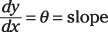##### Mechanics of Materials For Dummies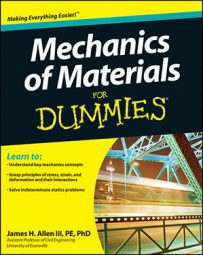Students and professional engineers in the mechanical sciences know that mechanics of materials deals extensively with stress on objects — from determining stress at a particular point to finding stresses in columns. Knowing how to apply some important laws and graphic representations can help you tackle stressful mechanics of materials problems with ease.

## Formulas for calculating stress at a point

When dealing with mechanics of materials, choosing the correct formula to calculate the stress at a given point can be difficult. Normal and shear stresses come in a wide variety of applications, each stress application with its own calculation formula. The most common stress types you deal with in basic mechanics of materials fall into several major categories:

• Axial tension: ANET is equal to the gross area of the cross section minus any holes that may exist.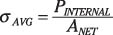• Thin-walled pressure vessels: Two stresses exist: an axial stress along the axis of the member and a hoop (or radial) stress, which occurs tangential to the radius of the cross section. These stresses are based on the gage pressure p inside the pressure vessel.

For cylindrical pressure vessels, use this pair of formulas: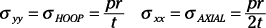For spherical pressure vessels, use the following formula: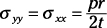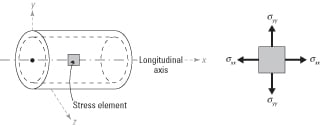• Bending stresses: For symmetric cross sections in the XY plane, use this formula: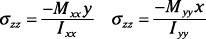• Flexural shear stresses: Here’s the formula for calculating flexural shear stress: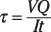• Torsional shear stress: Use this formula to find torsional shear stress: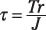## Computing stresses in columns

Knowing how to compute the stress in a column (compression member) is a basic point of knowledge in mechanics of materials. Determine if the column is ‘ short, slender, or intermediate by computing its maximum slenderness ratio (KL/r). For short columns, the stress of a member in compression is the basic axial stress formulation. For intermediate and slender columns, you can use the generalized Euler’s buckling equation. Approximate slenderness ratios for steel columns are shown in parentheses.

• Short columns: Slenderness ratio (KL/r < 50).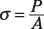• Slender columns: Slenderness ratio (KL/r ≥200). The calculation for slender columns uses the modulus of elasticity (E).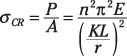• Intermediate columns: Slenderness ratio (50 ≤ KL/r < 200). The formula for intermediate columns uses the tangential modulus of elasticity (Et).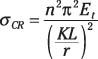## Using Mohr's circle to find principal stresses and angles

Anyone in the mechanical sciences is likely familiar with Mohrs circle — a useful graphical technique for finding principal stresses and strains in materials. Mohr’s circle also tells you the principal angles (orientations) of the principal stresses without your having to plug an angle into stress transformation equations.

Starting with a stress or strain element in the XY plane, construct a grid with a normal stress on the horizontal axis and a shear stress on the vertical. (Positive shear stress plots at the bottom.) Then just follow these steps:

1. Plot the vertical face coordinates V(σxx , τxy).

2. Plot the horizontal coordinates H(σyy, –τxy).

You use the opposite sign of the shear stress from Step 1 because the shear stresses on the horizontal faces are creating a couple that balances (or acts in the opposite direction of) the shear stresses on the vertical faces.

3. Draw a diameter line connecting Points V (from Step 1) and H (from Step 2).

4. Sketch the circle around the diameter from Step 3.

The circle should pass through Points V and H as shown here.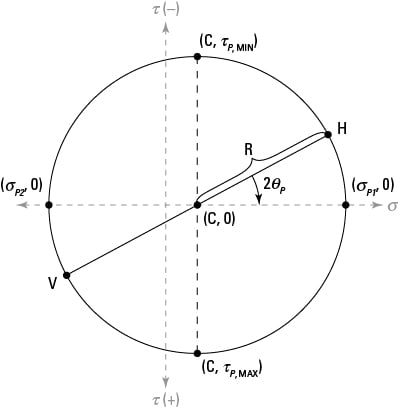5. Compute the normal stress position for the circle’s center point (C).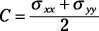6. Calculate the radius (R) for the circle.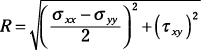7. Determine the principal stresses σP1 and σP2.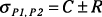8. Compute the principal angles ΘP1 and ΘP2.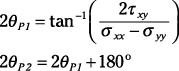You could also use equations directly (instead of Mohr’s circle) to determine transformed stresses at any angle: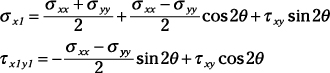To construct a Mohr’s circle for strain or to use the transformation equations, substitute εxx for σxx, εyy for σyy, and (0.5)γxy for τxy in the preceding

## Using a generalized Hooke's law for stress and strain

In mechanics of materials, Hookes law is the relationship that connects stresses to strains. Although Hooke’s original law was developed for uniaxial stresses, you can use a generalized version of Hooke’s law to connect stress and strain in three-dimensional objects, as well. Eventually, Hooke’s law helps you relate stresses (which are based on loads) to strains (which are based on deformations).

For a three-dimensional state of stress, the normal strain in a given direction (such as x) is a function of the stresses in all three orthogonal directions (usually the Cartesian x, y-, and z-directions), as shown by this equation: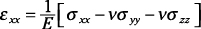where E is the modulus of elasticity and ν is Poisson’s ratio for the material. For a uniaxial stress, two of the stresses in the equation are zero. For a biaxial stress condition, one of the stresses in this equation is zero.

The generalized relationship for Hooke’s law for shear in the XY plane can be given as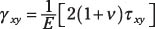Deformations measure a structure’s response under a load, and calculating that deformation is an important part of mechanics of materials. Deformation calculations come in a wide variety, depending on the type of load that causes the deformation. Axial deformations are caused by axial loads and angles of twist are causes by torsion loads. The elastic curve for flexural members is actually a differential equation.

The following list shows some of the most commonly used deformation expressions you encounter in mechanics of materials:

• Axial deformation: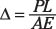• Angle of twist for torsion:• Double integrating to find deformations of beams:

You can approximate y(x), the equation of the elastic curve as a function of x, by the following differential equation: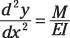You need to first find the generalized moment equation M at all locations along the beam as a function of position x. Solve this equation by integrating twice and applying boundary conditions to solve for constants of integration (known support displacements (y) and rotations (θ). Remember,